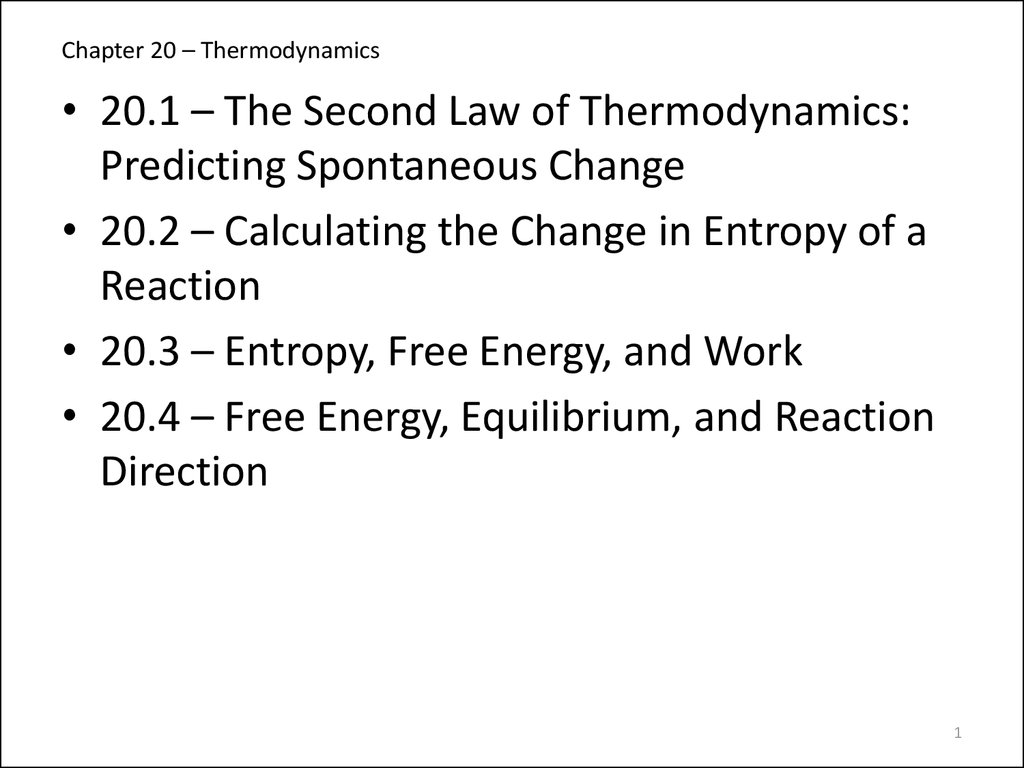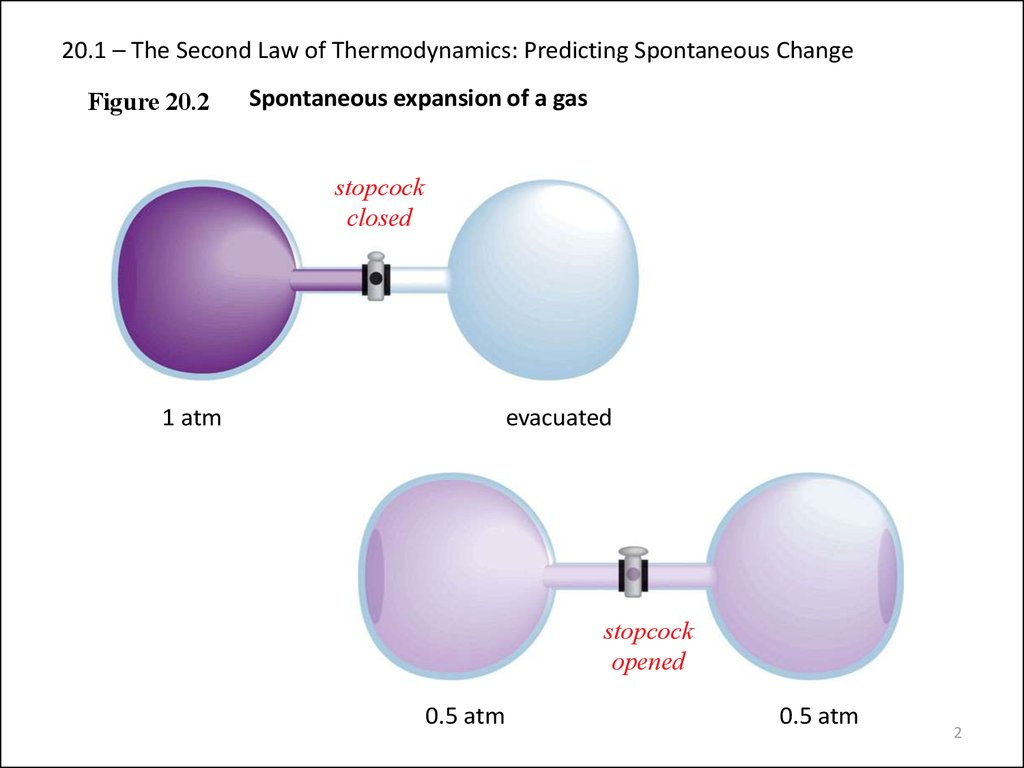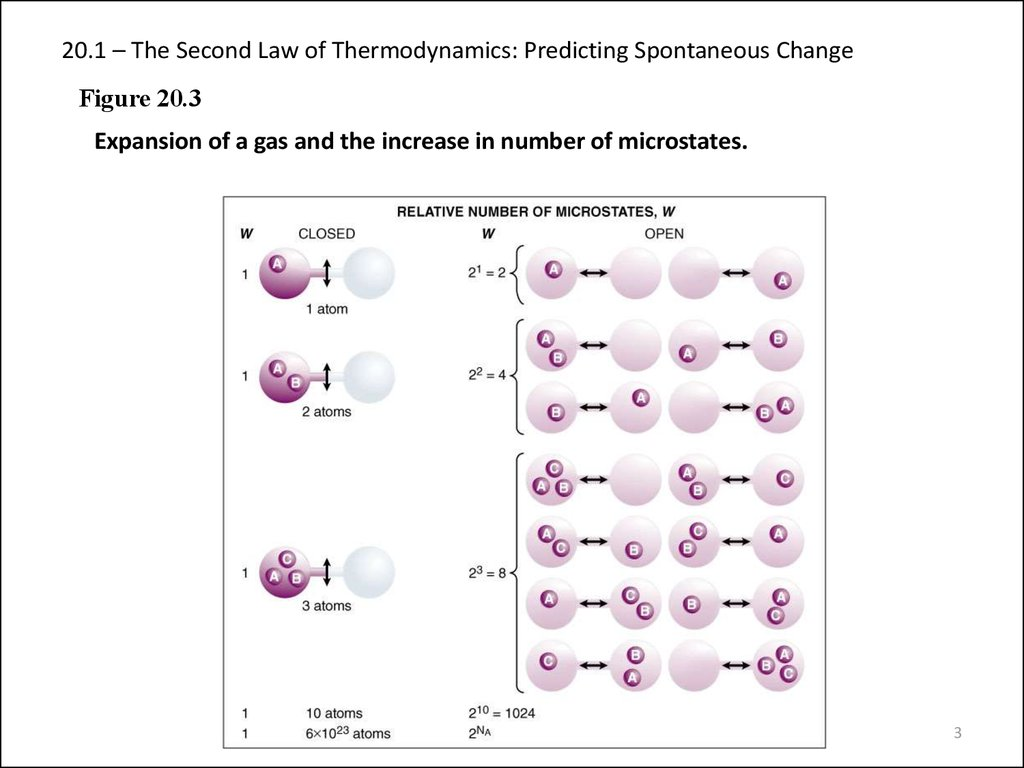# Chapter 20 Thermodynamics

## 1. Chapter 20 – Thermodynamics

• 20.1 – The Second Law of Thermodynamics:
Predicting Spontaneous Change
• 20.2 – Calculating the Change in Entropy of a
Reaction
• 20.3 – Entropy, Free Energy, and Work
• 20.4 – Free Energy, Equilibrium, and Reaction
Direction
1

## 2. 20.1 – The Second Law of Thermodynamics: Predicting Spontaneous Change

Figure 20.2
Spontaneous expansion of a gas
stopcock
closed
1 atm
evacuated
stopcock
opened
0.5 atm
0.5 atm
2

## 3. 20.1 – The Second Law of Thermodynamics: Predicting Spontaneous Change

Figure 20.3
Expansion of a gas and the increase in number of microstates.
3

## 4. 20.1 – The Second Law of Thermodynamics: Predicting Spontaneous Change

1877 Ludwig Boltzman
S = k ln W
where S is entropy, W is the number of ways of arranging the components of a
system, and k is a constant (the Boltzman constant), R/NA (R = universal gas constant,
•A system with relatively few equivalent ways to arrange its components (smaller
W) has relatively less disorder and low entropy.
•A system with many equivalent ways to arrange its components (larger W) has
relatively more disorder and high entropy.
DSuniverse = DSsystem + DSsurroundings > 0
The second law of thermodynamics.
4

## 5. 20.1 – The Second Law of Thermodynamics: Predicting Spontaneous Change

Figure 20.4
Random motion in a crystal
The third law of
thermodynamics.
A perfect crystal has zero
entropy at a temperature
of absolute zero.
Ssystem = 0 at 0 K
5

## 6. 20.1 – The Second Law of Thermodynamics: Predicting Spontaneous Change

Figure 20.5 The increase in entropy from solid to liquid to gas.
6

## 7. 20.1 – The Second Law of Thermodynamics: Predicting Spontaneous Change

Figure 20.6
The entropy change accompanying the dissolution of a salt.
pure solid
MIX
pure liquid
solution
7

## 8. 20.1 – The Second Law of Thermodynamics: Predicting Spontaneous Change

Figure 20.7
The small increase in entropy when ethanol dissolves in water.
Ethanol
Water
Solution of
ethanol and
water
8

## 9. 20.1 – The Second Law of Thermodynamics: Predicting Spontaneous Change

Figure 20.8
The large decrease in entropy when a gas dissolves in a liquid.
O2 gas
O2 gas in H2O
9

## 10. 20.1 – The Second Law of Thermodynamics: Predicting Spontaneous Change

Figure 20.9
Entropy and vibrational motion.
NO
NO2
N2O4
10

## 11. 20.1 – The Second Law of Thermodynamics: Predicting Spontaneous Change

Sample Problem 20.1:
PROBLEM:
Predicting Relative Entropy Values
Choose the member with the higher entropy in each of the following
pairs, and justify your choice [assume constant temperature, except in
part (e)]:
(a) 1mol of SO2(g) or 1mol of SO3(g)
(b) 1mol of CO2(s) or 1mol of CO2(g)
(c) 3mol of oxygen gas (O2) or 2mol of ozone gas (O3)
(d) 1mol of KBr(s) or 1mol of KBr(aq)
(e) Seawater in midwinter at 20C or in midsummer at 230C
(f) 1mol of CF4(g) or 1mol of CCl4(g)
PLAN:
In general less ordered systems have higher entropy than ordered
systems and entropy increases with an increase in temperature.
SOLUTION:
(a) 1mol of SO3(g) - more atoms
(d) 1mol of KBr(aq) - solution > solid
(b) 1mol of CO2(g) - gas > solid
(e) 230C - higher temperature
(c) 3mol of O2(g) - larger #mols
(f) CCl4 - larger mass
11

## 12. 20.2 – Calculating the Change in Entropy of a Reaction

Sample Problem 20.2:
PROBLEM:
Calculating the Standard Entropy of Reaction, DS0rxn
Calculate DS0rxn for the combustion of 1mol of propane at 250C.
C3H8(g) + 5O2(g)
PLAN:
3CO2(g) + 4H2O(l)
Use summation equations. It is obvious that entropy is being lost
because the reaction goes from 6 mols of gas to 3 mols of gas.
SOLUTION:
Find standard entropy values in the Appendix or other table.
DS = [(3 mol)(S0 CO2) + (4 mol)(S0 H2O)] - [(1 mol)(S0 C3H8) + (5 mol)(S0 O2)]
DS = [(3 mol)(213.7J/mol*K) + (4 mol)(69.9J/mol*K)] - [(1 mol)(269.9J/mol*K) + (5
mol)(205.0J/mol*K)]
DS = - 374 J/K
12

## 13. 20.2 – Calculating the Change in Entropy of a Reaction

Sample Problem 20.3:
PROBLEM:
Determining Reaction Spontaneity
At 298K, the formation of ammonia has a negative DS0sys;
N2(g) + 3H2(g)
2NH3(g)
DS0sys = -197 J/K
Calculate DS0rxn, and state whether the reaction occurs
spontaneously at this temperature.
PLAN:
DS0universe must be > 0 in order for this reaction to be spontaneous, so
DS0surroundings must be > 197 J/K. To find DS0surr, first find DHsys; DHsys = DHrxn
which can be calculated using DH0f values from tables. DS0universe = DS0surr +
DS0sys.
SOLUTION:
DH0rx = [(2 mol)(DH0fNH3)] - [(1 mol)(DH0fN2) + (3 mol)(DH0fH2)]
DH0rx = -91.8 kJ
DS0surr = -DH0sys/T =
-(-91.8x103J/298K)
DS0universe = DS0surr + DS0sys
= 308 J/K
= 308 J/K + (-197 J/K) = 111 J/K
DS0universe > 0 so the reaction is spontaneous.
13

## 14. 20.3 – Entropy, Free Energy, and Work

Sample Problem 20.4:
PROBLEM:
Calculating DG0 from Enthalpy and Entropy Values
Potassium chlorate, a common oxidizing agent in fireworks and
matchheads, undergoes a solid-state disproportionation reaction when
heated.
Note that the oxidation number of Cl in the reactant is higher in one of the products and
lower in the other (disproportionation).
+5
4KClO3(s)
D
+7
-1
3KClO4(s) + KCl(s)
Use DH0f and S0 values to calculate DG0sys (DG0rxn) at 250C for this reaction.
PLAN:
Use Appendix B values for thermodynamic entities; place them into the
Gibbs Free Energy equation and solve.
SOLUTION:
DH0rxn = S mDH0products - S nDH0reactants
DH0rxn = (3 mol)(-432.8 kJ/mol) + (1 mol)(-436.7 kJ/mol) (4 mol)(-397.7 kJ/mol)
DH0rxn = -144 kJ
14

## 15. 20.3 – Entropy, Free Energy, and Work

Sample Problem 20.4:
Calculating DG0 from Enthalpy and Entropy Values
continued
DS0rxn = S mDS0products - S nDS0reactants
DS0rxn = (3 mol)(151 J/mol*K) + (1 mol)(82.6 J/mol*K) (4 mol)(143.1 J/mol*K)
DS0rxn = -36.8 J/K
DG0rxn = DH0rxn - T DS0rxn
DG0rxn = -144 kJ - (298K)(-36.8 J/K)(kJ/103 J)
DG0rxn = -133 kJ
15

## 16. 20.3 – Entropy, Free Energy, and Work

Sample Problem 20.5:
PROBLEM:
PLAN:
Calculating DG0rxn from DG0f Values
Use DG0f values to calculate DGrxn for the reaction in Sample
Problem 20.4:
D
4KClO3(s)
3KClO4(s) + KCl(s)
Use the DG summation equation.
SOLUTION:
DG0rxn = S mDG0products - S nDG0reactants
DG0rxn = (3mol)(-303.2kJ/mol) + (1mol)(-409.2kJ/mol) (4mol)(-296.3kJ/mol)
DG0rxn = -134kJ
16

## 17. 20.3 – Entropy, Free Energy, and Work

Sample Problem 20.6:
PROBLEM:
Determining the Effect of Temperature on DG0
An important reaction in the production of sulfuric acid is the
oxidation of SO2(g) to SO3(g):
2SO2(g) + O2(g)
2SO3(g)
At 298K, DG0 = -141.6kJ; DH0 = -198.4kJ; and DS0 = -187.9J/K
(a) Use the data to decide if this reaction is spontaneous at 250C, and predict how
DG0 will change with increasing T.
(b) Assuming DH0 and DS0 are constant with increasing T, is the reaction spontaneous
at 900.0C?
PLAN:
SOLUTION:
The sign of DG0 tells us whether the reaction is spontaneous and the
signs of DH0 and DS0 will be indicative of the T effect. Use the Gibbs
free energy equation for part (b).
(a) The reaction is spontaneous at 250C because DG0 is (-). Since
DH0 is (-) but DS0 is also (-), DG0 will become less spontaneous as
the temperature increases.
17

## 18. 20.3 – Entropy, Free Energy, and Work

Sample Problem 20.6:
Determining the Effect of Temperature on DG0
continued
(b) DG0rxn = DH0rxn - T DS0rxn
DG0rxn = -198.4kJ - (1173K)(-187.9J/mol*K)(kJ/103J)
DG0rxn = 22.0 kJ; the reaction will be nonspontaneous at 900.0C
18

## 19. 20.3 – Entropy, Free Energy, and Work

Figure B20.3
The coupling of a nonspontaneous reaction to the hydrolysis of ATP.
19

## 20. 20.3 – Entropy, Free Energy, and Work

Figure B20.4 The cycling of metabolic free enery through ATP
20

## 21. 20.3 – Entropy, Free Energy, and Work

Figure B20.5
Why is ATP a high-energy molecule?
21

## 22.

20.4 – Free Energy, Equilibrium, and Reaction Direction
Free Energy, Equilibrium and Reaction Direction
•If Q/K < 1, then ln Q/K < 0; the reaction proceeds to the right (DG < 0)
•If Q/K > 1, then ln Q/K > 0; the reaction proceeds to the left (DG > 0)
•If Q/K = 1, then ln Q/K = 0; the reaction is at equilibrium (DG = 0)
DG = RT ln Q/K = RT lnQ - RT lnK
Under standard conditions (1M concentrations, 1atm for gases), Q = 1 and ln
Q = 0 so
DG0 = - RT lnK

## 23.

20.4 – Free Energy, Equilibrium, and Reaction Direction
Table 20.2 The Relationship Between DG0 and K at 250C
DG0(kJ)
K
100
3x10-18
50
2x10-9
10
2x10-2
1
7x10-1
0
1
-1
1.5
-10
5x101
-50
6x108
-100
3x1017
-200
1x1035
Essentially no forward reaction; reverse
reaction goes to completion
Forward and reverse reactions proceed to
same extent
Forward reaction goes to completion;
essentially no reverse reaction
REVERSE REACTION
9x10-36
FORWARD REACTION
200
Significance

## 24.

20.4 – Free Energy, Equilibrium, and Reaction Direction
Sample Problem 20.7:
PROBLEM:
Calculating DG at Nonstandard Conditions
The oxidation of SO2, which we considered in Sample Problem 20.6
2SO2(g) + O2(g)
2SO3(g)
is too slow at 298K to be useful in the manufacture of sulfuric acid. To overcome this
low rate, the process is conducted at an elevated temperature.
(a) Calculate K at 298K and at 973K. (DG0298 = -141.6kJ/mol of reaction as written
using DH0 and DS0 values at 973K. DG0973 = -12.12kJ/mol of reaction as written.)
(b) In experiments to determine the effect of temperature on reaction spontaneity,
two sealed containers are filled with 0.500atm of SO2, 0.0100atm of O2, and
0.100atm of SO3 and kept at 250C and at 700.0C. In which direction, if any, will the
reaction proceed to reach equilibrium at each temperature?
(c) Calculate DG for the system in part (b) at each temperature.

## 25.

20.4 – Free Energy, Equilibrium, and Reaction Direction
Sample Problem 20.7:
Calculating DG at Nonstandard Conditions
continued (2 of 3)
SOLUTION:
DG0
(a) Calculating K at the two temperatures:
= -RTlnK so
(DG / RT)
K e
At 298, the exponent is -DG0/RT
(DG / RT)
K e
0
= e57.2
0
=-
K e
0
= e1.50
(8.314J/mol*K)(298K)
= 57.2
= 7x1024
(-12.12kJ/mol)(103J/kJ)
At 973, the exponent is -DG0/RT
(DG / RT)
(-141.6kJ/mol)(103J/kJ)
(8.314J/mol*K)(973K)
= 4.5
= 1.50

## 26.

20.4 – Free Energy, Equilibrium, and Reaction Direction
Sample Problem 20.7:
Calculating DG at Nonstandard Conditions
continued (3 of 3)
(b) The value of Q =
pSO32
(pSO2)2(pO2)
(0.100)2
=
(0.500)2(0.0100)
= 4.00
Since Q is < K at both temperatures the reaction will shift right; for 298K there
will be a dramatic shift while at 973K the shift will be slight.
(c) The nonstandard DG is calculated using DG = DG0 + RTlnQ
DG298 = -141.6kJ/mol + (8.314J/mol*K)(kJ/103J)(298K)(ln4.00)
DG298 = -138.2kJ/mol
DG973 = -12.12kJ/mol + (8.314J/mol*K)(kJ/103J)(973K)(ln4.00)
DG298 = -0.9kJ/mol